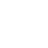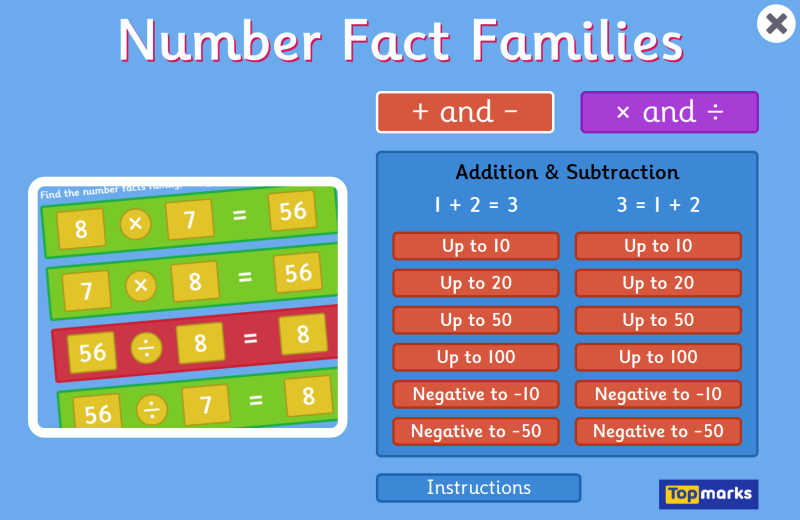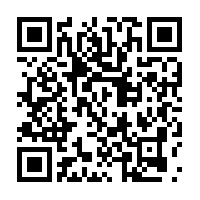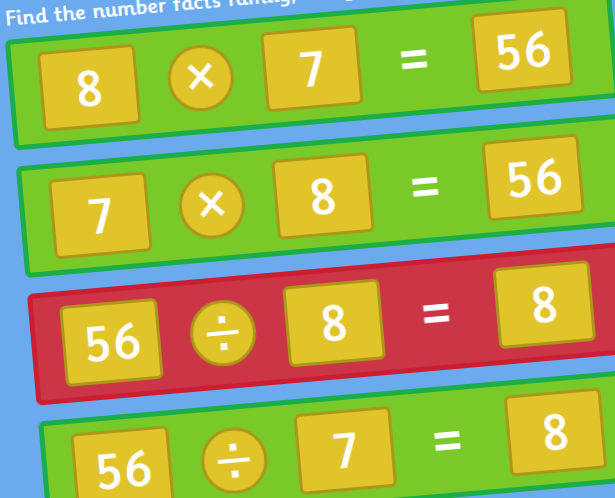# Number Fact Families## QR CODE

Scan this on mobiles and tablets to quickly open this web page.Number Fact Families is a maths game where you need to work out the collection of related addition and subtraction or multiplication and division fact family relationships given 3 randomised numbers. Recognising fact families is an important number concept where you can recognise inverse operations such as 3 + 5 = 8 and 8 - 3 = 5.

This game has differing levels of difficulty including challenging equations involving negative numbers.

AGE
6-11 Years
SUBJECTS
Maths Number Addition Subtraction Multiplication Division# Number Fact Families• + and -
• × and ÷

## 1 + 2 = 3

• Up to 10
• Up to 20
• Up to 50
• Up to 100
• Negative to -10
• Negative to -50

## 3 = 1 + 2

• Up to 10
• Up to 20
• Up to 50
• Up to 100
• Negative to -10
• Negative to -50

• 2 to 5
Times Tables
• 6 to 12
Times Tables
• 2 to 12
Times Tables

## 8 = 2 × 4

• 2 to 5
Times Tables
• 6 to 12
Times Tables
• 2 to 12
Times Tables